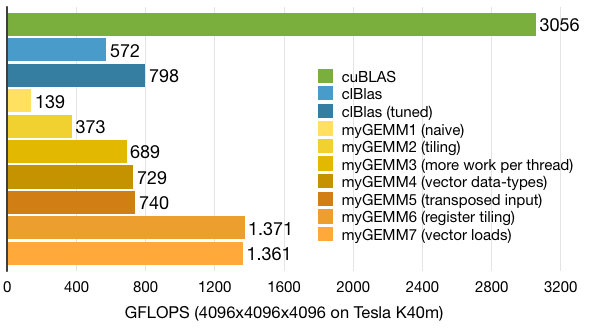# Tutorial: OpenCL SGEMM tuning for Kepler

Note: the complete source-code is available at GitHub.
Note2: a tuned OpenCL BLAS library based on this tutorial is now available at GitHub.
Note3: a WebGL2 demo of this tutorial is available at: https://www.ibiblio.org/e-notes/webgl/gpu/mul/sgemm.htm.

## Kernel 7: Wider loads with register blocking

In one of our earlier kernels we used wider data-types to get 64-bit or 128-bit loads and stores. Let's re-integrate that in our latest version with 2D register blocking. We'll do this for loads only.

The launch configuration and the constants remain the same. The kernel is also quite similar, with the main exceptions in the part where we are loading from off-chip memory:

• We now load float2 or float4 values from off-chip memory. Therefore, the loop over the amount of loads per thread has been reduced by a factor WIDTH. The same holds for the indexing into the vector arrays.
• Since we now have a slightly different access pattern when storing data into Asub and Bsub, we choose to swap the column and row indices of Bsub back to how they are for Asub. This does require more local memory loads (LDS instead of LDS.64) in the computational loop, but since this is now cached using register tiling, its impact is not that big. The main new advantage is that we can now also store data into Bsub using vector data-types. Note that since Asub and Bsub are not declared as vectors, the stores into Asub and Bsub look scalar from a coding-perspective, but they become 64-bit or 128-bit stores (STS.128) when compiled. We can now also remove the padding, giving us that extra bit of local memory back.

Note that we still have the restriction that TSM has to be equal to TSN. This restriction can be removed by creating two loops when loading, one over LPTA with indices constrained to TSM and one over LPTB with indices constrained to TSN. We'll implement this in our final version.

```// Wider loads combined with 2D register blocking
__kernel void myGEMM7(const int M, const int N, const int K,
const __global floatX* A,
const __global floatX* B,
__global float* C) {

const int tidm = get_local_id(0); // Local row ID (max: TSM/WPTM)
const int tidn = get_local_id(1); // Local col ID (max: TSN/WPTN)
const int offsetM = TSM*get_group_id(0); // Work-group offset
const int offsetN = TSN*get_group_id(1); // Work-group offset

// Local memory to fit a tile of A and B
__local float Asub[TSK][TSM];
__local float Bsub[TSK][TSN];

// Allocate register space
float Areg;
float Breg[WPTN];
float acc[WPTM][WPTN];

// Initialise the accumulation registers
for (int wm=0; wm<WPTM; wm++) {
for (int wn=0; wn<WPTN; wn++) {
acc[wm][wn] = 0.0f;
}
}

// Loop over all tiles
int numTiles = K/TSK;
for (int t=0; t<numTiles; t++) {

// Load one tile of A and B into local memory
for (int la=0; la<LPTA/WIDTH; la++) {
int tid = tidn*RTSM + tidm;
int id = la*RTSN*RTSM + tid;
int row = id % (TSM/WIDTH);
int col = id / (TSM/WIDTH);

int tiledIndex = TSK*t + col;
floatX vecA = A[tiledIndex*(M/WIDTH) + offsetM/WIDTH + row];
floatX vecB = B[tiledIndex*(N/WIDTH) + offsetN/WIDTH + row];

// Store the loaded vectors into local memory
#if WIDTH == 1
Asub[col][row] = vecA;
Asub[col][row] = vecA;
#elif WIDTH == 2
Asub[col][WIDTH*row + 0] = vecA.x;
Asub[col][WIDTH*row + 1] = vecA.y;
#elif WIDTH == 4
Asub[col][WIDTH*row + 0] = vecA.x;
Asub[col][WIDTH*row + 1] = vecA.y;
Asub[col][WIDTH*row + 2] = vecA.z;
Asub[col][WIDTH*row + 3] = vecA.w;
#endif
#if WIDTH == 1
Bsub[col][row] = vecB;
Bsub[col][row] = vecB;
#elif WIDTH == 2
Bsub[col][WIDTH*row + 0] = vecB.x;
Bsub[col][WIDTH*row + 1] = vecB.y;
#elif WIDTH == 4
Bsub[col][WIDTH*row + 0] = vecB.x;
Bsub[col][WIDTH*row + 1] = vecB.y;
Bsub[col][WIDTH*row + 2] = vecB.z;
Bsub[col][WIDTH*row + 3] = vecB.w;
#endif
}

// Synchronise to make sure the tile is loaded
barrier(CLK_LOCAL_MEM_FENCE);

// Loop over the values of a single tile
for (int k=0; k<TSK; k++) {

// Cache the values of Bsub in registers
for (int wn=0; wn<WPTN; wn++) {
int col = tidn + wn*RTSN;
Breg[wn] = Bsub[k][col];
}

// Perform the computation
for (int wm=0; wm<WPTM; wm++) {
int row = tidm + wm*RTSM;
Areg = Asub[k][row];
for (int wn=0; wn<WPTN; wn++) {
acc[wm][wn] += Areg * Breg[wn];
}
}
}

barrier(CLK_LOCAL_MEM_FENCE);
}

// Store the final results in C
for (int wm=0; wm<WPTM; wm++) {
int globalRow = offsetM + tidm + wm*RTSM;
for (int wn=0; wn<WPTN; wn++) {
int globalCol = offsetN + tidn + wn*RTSN;
C[globalCol*M + globalRow] = acc[wm][wn];
}
}
}
```

After inspection of the assembly code, we see that we went from 16 32-bit loads (LD) and local stores (STS) to 4 128-bit loads (LD.128) and local stores (STS.128) for a vector width of 4 floats.

Performance with WIDTH equal to 4 is slightly worse compared to our previous kernel. As before, this is because everything is very sensitive to register pressure and compiler optimisations. Luckily these wider loads will be paying off for the next couple of kernels, so we'll definitely keep them in.Tutorial written by Cedric Nugteren, (c) 2014 SURFsara
Last updated in 2014 - information might be outdated